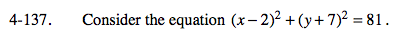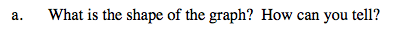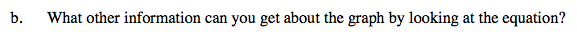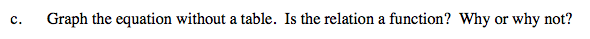### Home > A2C > Chapter 4 > Lesson 4.3.1 > Problem4-137

4-137.What family of equations does this look like?

A circle looks like the family (xh)2+(yk)2 = r 2.What is the center of the circle? What is the value of the radius?To graph a circle, all you need to know is its center point and its radius. How do you find these?

In this case, the center of the circle is not at the origin. The center of the circle will be moved two to the right and seven down, located at the point (2, −7). The radius, in this case, is the square root of 81.

What is the definition of a function? Is this graph consistent with the definition?